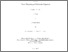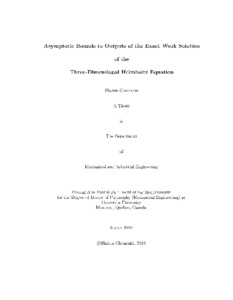Title:

# Asymptotic bounds to outputs of the exact weak solution of the three-dimensional Helmholtz equation

Ghomeshi, Shahin (2010) Asymptotic bounds to outputs of the exact weak solution of the three-dimensional Helmholtz equation. PhD thesis, Concordia University.Preview
Text (application/pdf)
NR80163.pdf - Accepted Version
4MB

## Abstract

In engineering practice, the design is based on certain design quantities or "outputs of interest" which are functionals of field variables such as displacement, velocity field, or pressure. In order to gain confidence in the numerical approximation of "outputs," a method of obtaining sharp, rigorous upper and lower bounds to outputs of the exact solution have been developed for symmetric and coercive problems (the Poisson equation and the elasticity equation), for non-symmetric coercive problems (advection-diffusion-reaction equation), and more recently for certain constrained problems (Stokes equation). In this thesis we develop the method for the Helmholtz equation. The common approach relies on decomposing the global problem into independent local elemental sub-problems by relaxing the continuity along the edges of a partitioning of the entire domain, using approximate Lagrange multipliers. The method exploits the Lagrangian saddle point property by recasting the output problem as a constrained minimization problem. The upper and lower computed bounds then hold for all levels of refinement and are shown to approach the exact solution at the same rate as its underlying finite element approach. The certificate of precision can then determine the best as well as the worst case scenario in an engineering design problem. This thesis addresses bounds to outputs of interest for the complex Helmholtz equation. The Helmholtz equation is in general non-coercive for high wave numbers and therefore, the previous approaches that relied on duality theory of convex minimization do not directly apply. Only in the asymptotic regime does the Helmholtz equation become coercive, and reliable (guaranteed) bounds can thus be obtained. Therefore, in order to achieve good bounds, several new ingredients have been introduced. The bounds procedure is firstly formulated with appropriate extension to complex-valued equations. Secondly, in the computation of the inter-subdomain continuity multipliers we follow the FETI-H approach and regularize the system matrix with a complex term to make the system non-singular. Finally, in order to obtain sharper output bounds in the presence of pollution errors, higher order nodal spectral element method is employed which has several computational advantages over the traditional finite element approach. We performed verification of our results and demonstrate the bounding properties for the Helmholtz problem.

Divisions: Concordia University > Gina Cody School of Engineering and Computer Science > Mechanical and Industrial Engineering Thesis (PhD) Ghomeshi, Shahin xiii, 141 leaves : ill. ; 29 cm. Concordia University Ph. D. Mechanical and Industrial Engineering 2010 Paraschivoiu, M LE 3 C66M43P 2010 G46 979321 Concordia University Library 09 Dec 2014 17:57 13 Jul 2020 20:12 https://concordiauniversity.on.worldcat....
All items in Spectrum are protected by copyright, with all rights reserved. The use of items is governed by Spectrum's terms of access.

Repository Staff Only: item control pageResearch related to the current document (at the CORE website)
Back to top